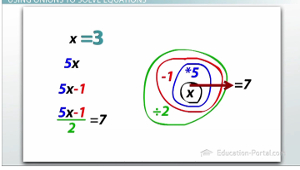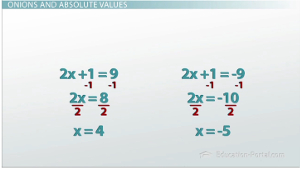# Solving Absolute Value Practice Problems

An error occurred trying to load this video.

Try refreshing the page, or contact customer support.

Coming up next: How to Graph an Absolute Value and Do Transformations

### You're on a roll. Keep up the good work!

Replay
Your next lesson will play in 10 seconds
• 0:10 Review of Absolute Values
• 1:06 Using Onions to Solve…
• 3:22 Onions and Absolute Values
• 4:39 Onions and Complex…
• 6:30 Lesson Summary

Want to watch this again later?

Timeline
Autoplay
Autoplay
Speed

#### Recommended Lessons and Courses for You

Lesson Transcript
Instructor: Zach Pino
There are many easy mistakes to make when solving absolute value equations. Learn how to avoid those mistakes here by working on examples of absolute value equations with operations on the inside and the outside of the absolute value.

## Review of Absolute ValuesWhen it comes to solving absolute value equations, the one most important thing to remember is that they always give you two answers. We get those two answers by splitting the equation up, but how and when to split it up is where it starts to get a little more complicated and also where the mistakes start happening. In order to avoid those mistakes, it really helps to have a conceptual understanding of what 'solving an equation' means.

So let's first ask ourselves a question that you probably don't hear every day; what does a mathematical equation have in common with an onion? Now, I suppose your answer could be 'they both make you cry,' but if that was your answer, I hope this video can change your mind. Well, just like an onion has a very center and then a bunch of layers on the outside, an equation has a very center (the number) and then a bunch of operations being done to that number.

## Using Onions to Solve Equations

So for example, an equation might tell us that we start with some number (lets call it x), we multiply it by 5, then we subtract 1, then we divide all of that by 2, and after we've done that, we apparently end up with 7. This can be expressed as a normal mathematical equation, or using this onion analogy where the very middle of the onion is the number we start with and each thing we do to that number is a new layer on the outside. So when we're asked to 'solve' this equation, it's simply our job to figure out what was that number in the very beginning that made it that after we do all these things we end up with 7. It's our job to peel off the layers of the onion one at a time to work our way all the way back to the very center - the x.Well the first layer I peel off is this divided by 2. After I divide it by 2, apparently I ended up with 7, which means that before I divided by 2, I must have had 14 because 14 divided by 2 is 7. So the way I can undo division is by multiplication because those are inverse operations. So by putting the little times 2 by this divide by 2, they undo each other - they cancel each other out - and over on the other side of the equation, I get 7 times 2 and I get 14. So that last step has been undone and now I have an equation with one less step.

The next layer I need to undo is the minus 1. We can undo subtraction with addition and by adding 1 to both sides of my equation, I can end up with 5 times x equals 15. Now the last layer I need to undo is multiplication by 5; I can undo multiplication with division. Those things undo and I'm left with 15 divided by 5, which is 3. This means that if we would have substituted 3 back into the very beginning and done all those different things to it, we would have ended up with 7.

## Onions and Absolute Values

Absolute value equations are done the exact same way. Le'ts take a look at the example |2x+1| = 9. If we draw our onion analogy, we start with a number (x), we multiply that by 2, then we add 1, and after we've done those things, we take the absolute value and we end up with 9.

To unlock this lesson you must be a Study.com Member.

### Register to view this lesson

Are you a student or a teacher?

### Unlock Your Education

#### See for yourself why 30 million people use Study.com

##### Become a Study.com member and start learning now.
Back
What teachers are saying about Study.com

### Earning College Credit

Did you know… We have over 160 college courses that prepare you to earn credit by exam that is accepted by over 1,500 colleges and universities. You can test out of the first two years of college and save thousands off your degree. Anyone can earn credit-by-exam regardless of age or education level.